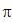CS 270 Examples of Well-Known Series
1) Geometric Series: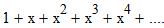=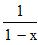converges for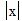<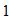2) Trigonometric Functions: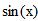=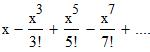converges for all x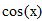=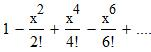converges for all x
3) Exponential and Logarithmic Functions: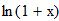=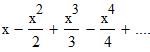converges for -1 < x <= 1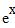=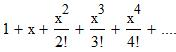converges for all x
4) Harmonic Series: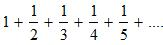=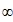That is, this series diverges.
5) Calculations with Series:
a) Term-by-term differentiation of the series for sin(x) produces the series for cos(x).
Question: When is term-by-term differentiation legal?
b) An interesting manipulation of a series:=and make the substitution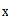=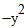This gives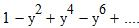=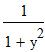Question: Is this legal?
Then integrate both sides from 0 to 1. Assuming that it is OK to integrate term-
by-term, we get: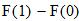=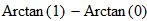where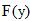=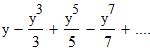Thus we get: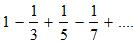=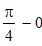Hence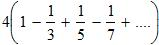=